Test: Quantitative Techniques- 9

# Test: Quantitative Techniques- 9

Test Description

## 20 Questions MCQ Test IBPS PO Prelims- Study Material, Online Tests, Previous Year | Test: Quantitative Techniques- 9

Test: Quantitative Techniques- 9 for Banking Exams 2023 is part of IBPS PO Prelims- Study Material, Online Tests, Previous Year preparation. The Test: Quantitative Techniques- 9 questions and answers have been prepared according to the Banking Exams exam syllabus.The Test: Quantitative Techniques- 9 MCQs are made for Banking Exams 2023 Exam. Find important definitions, questions, notes, meanings, examples, exercises, MCQs and online tests for Test: Quantitative Techniques- 9 below.
Solutions of Test: Quantitative Techniques- 9 questions in English are available as part of our IBPS PO Prelims- Study Material, Online Tests, Previous Year for Banking Exams & Test: Quantitative Techniques- 9 solutions in Hindi for IBPS PO Prelims- Study Material, Online Tests, Previous Year course. Download more important topics, notes, lectures and mock test series for Banking Exams Exam by signing up for free. Attempt Test: Quantitative Techniques- 9 | 20 questions in 30 minutes | Mock test for Banking Exams preparation | Free important questions MCQ to study IBPS PO Prelims- Study Material, Online Tests, Previous Year for Banking Exams Exam | Download free PDF with solutions
 1 Crore+ students have signed up on EduRev. Have you?
Test: Quantitative Techniques- 9 - Question 1

### 121,225,361, ____? _____

Detailed Solution for Test: Quantitative Techniques- 9 - Question 1

121  225  361  ?

112  152  192  233

So ?  =  529

Test: Quantitative Techniques- 9 - Question 2

### A man is six times as old as his son. Four years hence, the sum of their ages will be 43 years. How old is his son now?

Detailed Solution for Test: Quantitative Techniques- 9 - Question 2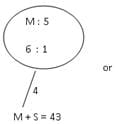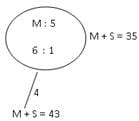So 7 ≡ 35
1 ≡ 5
So age of son = 5 years

Test: Quantitative Techniques- 9 - Question 3

### Arun can climb a coconut tree by 1.5 feet by each lift; however he slips 0.5 feets every time he makes the next lift. How many lifts he will have to take to reach the top of the coconut tree. If height of tree is 18.5 feet?

Detailed Solution for Test: Quantitative Techniques- 9 - Question 3

Height covered in 17 lifts = (1.5 - .05) x 17 = 17 feet
Height covered in 18th lift = 1.5

Test: Quantitative Techniques- 9 - Question 4

Jogen’s taxable income for 2010-11 is rs.5,00,000. Tax rates are 1. Nil for 1st 150000; 2. 10% for 150000-300000 and 20% for the remaining. his tax liability is?

Detailed Solution for Test: Quantitative Techniques- 9 - Question 4

Total tax = (1,50,000) x 0 + 1,50,000 x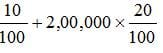⇒ 55, 000

Test: Quantitative Techniques- 9 - Question 5

If A is 40% more than B and B is 20% less than C then A:C is?

Detailed Solution for Test: Quantitative Techniques- 9 - Question 5

A  :  B  :  C

112   80   100
So   A : C ⇒ 28:25

Test: Quantitative Techniques- 9 - Question 6

The ratio of income of A and B is 7:3 and that of their expenditure is 5:2. If both of them save Rs. 300 each. Then find the income of A?

Detailed Solution for Test: Quantitative Techniques- 9 - Question 6

A  B
Income   7   :  3
Income 21 : 9
or
Expenditure   5  :  2
Exp  20 : 8
sav  1 : 1
1 ≡ 300
So   21 = 6300
Income of A = 6300

Test: Quantitative Techniques- 9 - Question 7

Original price of TV set is Rs. 9000. The price is discounted by 20% and then raised by10%. What is the new price?

Detailed Solution for Test: Quantitative Techniques- 9 - Question 7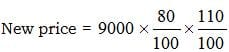= 7920

Test: Quantitative Techniques- 9 - Question 8

A student walks from his house at 4km/hr and reaches his school 5 mins too late, if his speed had been 5km/hr he would have reached 10 minutes too early. How far is the school from his house?

Detailed Solution for Test: Quantitative Techniques- 9 - Question 8

Let the distance between school and home be :- x km

The difference of time when A goes house to school with these tw different speed is 15 min.

=> 15 / 60 hour.

Therefore;

Now,

d/4 - d/5 = 15/60

5d - 4d/20  =  15/60

d/20 =  1/4

=>  d = 5 km

Test: Quantitative Techniques- 9 - Question 9

Find the least number of 5 digits which is exactly divisible by 129?

Detailed Solution for Test: Quantitative Techniques- 9 - Question 9

The correct option is A.
The smallest 5-digit number exactly divisible by 129 is 10,062.
129 X 78 = 10,062

Test: Quantitative Techniques- 9 - Question 10

Find the average of 1st seven prime numbers?

Detailed Solution for Test: Quantitative Techniques- 9 - Question 10

Average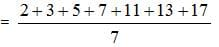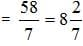Test: Quantitative Techniques- 9 - Question 11

What must be added to each term of ratio 7:11, so as to make it equal to 3:4?

Test: Quantitative Techniques- 9 - Question 12

In a group of five boys and five girls, 3 persons are to be selected for a competition. What is the probability that all three persons are girls?

Detailed Solution for Test: Quantitative Techniques- 9 - Question 12

Required Probability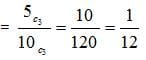Test: Quantitative Techniques- 9 - Question 13

Two coins are tossed simultaneously. The probability of getting at most one tail is?

Test: Quantitative Techniques- 9 - Question 14

A flag pole 18m high castes a shadow 9.6m long. What is the distance of the top of the pole from the end of the shadow?

Detailed Solution for Test: Quantitative Techniques- 9 - Question 14

Given , Flag height = 18 m
Distance between top of flag and end of shadow = x2 = 182 + 9.62
= 324+92.16
= 416.16
= (20.4)2
x = 20.4m

Test: Quantitative Techniques- 9 - Question 15

A box contains 2 red balls, 3 blue balls and 4 black balls. 3 balls are drawn at random. Find the probability that all balls are blue?

Detailed Solution for Test: Quantitative Techniques- 9 - Question 15

Required Probability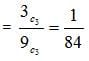Test: Quantitative Techniques- 9 - Question 16

A copper sphere of diameter 18cm is drawn into a wire of diameter 4mm. Find the length of the wire (in meter)?

Detailed Solution for Test: Quantitative Techniques- 9 - Question 16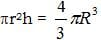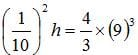h = 24,300 cm
So h = 243 meter

Test: Quantitative Techniques- 9 - Question 17

What is the circumference of wheel whose radius is 14cm ?

Detailed Solution for Test: Quantitative Techniques- 9 - Question 17

Circumference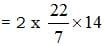= 88

Test: Quantitative Techniques- 9 - Question 18

6156÷√y × 53 = 4028

Detailed Solution for Test: Quantitative Techniques- 9 - Question 18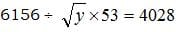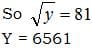Test: Quantitative Techniques- 9 - Question 19

A dishonest dealer sys that he sells his goods at C.P, but he weighs 900gm for a kg. What is his gain %?

Detailed Solution for Test: Quantitative Techniques- 9 - Question 19

Required gain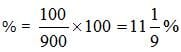Test: Quantitative Techniques- 9 - Question 20

The area of a square park is 25km2. The time taken to complete a round of the field once, at a speed of 3km/hr?

Detailed Solution for Test: Quantitative Techniques- 9 - Question 20

Area = 25 Km2
Side = 5 Km
Perimeter = 4 x 5 = 20 Km
Time = 20/3=6h 40min

## IBPS PO Prelims- Study Material, Online Tests, Previous Year

173 videos|192 docs|149 tests
 Use Code STAYHOME200 and get INR 200 additional OFF Use Coupon Code
Information about Test: Quantitative Techniques- 9 Page
In this test you can find the Exam questions for Test: Quantitative Techniques- 9 solved & explained in the simplest way possible. Besides giving Questions and answers for Test: Quantitative Techniques- 9, EduRev gives you an ample number of Online tests for practice

## IBPS PO Prelims- Study Material, Online Tests, Previous Year

173 videos|192 docs|149 tests